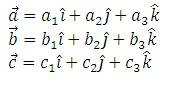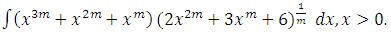Click to Chat

1800-1023-196

+91-120-4616500

CART 0

• 0

MY CART (5)

Use Coupon: CART20 and get 20% off on all online Study Material

ITEM
DETAILS
MRP
DISCOUNT
FINAL PRICE
Total Price: Rs.

There are no items in this cart.
Continue Shopping• Complete JEE Main/Advanced Course and Test Series
• OFFERED PRICE: Rs. 15,900
• View Details

```IIT-JEE-Mathematics-Mains-2002

Mains

Time : Two hours                                                                  Max. Marks : 60
______________________________________________________________________

Instructions

1.     The Question Paper has 4 printed pages and has 12 questions. Please ensure that the copy of the Question Paper you have received contains all the questions.

2.     Attempts ALL questions. Each question carries 5 marks.

3.     Answer each question starting on a new page. The corresponding Question number must be written in the left margin.

4.     Do not write more than one answers for the same question. In case you attempt a question more than once, please cancel the answer(s) you consider to be wrong. Otherwise, only the answer appearing last will be evaluated.

5.     Use only Arabic numerals (0, 1, 2 .............9) in answering the questions irrespective of the language in which your answer.

6.     Use of logarithmic tables is NOT PERMITTED.

7.     Use of calculators is NOT PERMITTED.

8.     Both magnitude and direction of vector quantities, if any, in your answer should be indicated clearly.

9.     Irrational numbers, if any, in your answer need not be expressed in decimal form.

10.  If the final answer appears in the form of a ratio or a product of two numbers, that need not to be further simplified.
_______________________________________________________________________

1.         Let a, b be positive real numbers. If a, A1, A2, be are in arithmetic progression, a, G1, G2, b are in geometric progression and a, H1, H2, b are in harmonic progression, show that

(G1 G2)/(H1 H2 ) = (A1+A2)/(H1+H2 ) = ((2a+b)(a+2b))/9ab

2.         Use mathematical induction to show that (25)n+1 - 24n + 5735 is divisible by (24)2 for all n = 1, 2

3.         Prove that cos tan-1 sin cot-1 x = √((x2+1)/(x2 +2))..

4.         A box contains N coins, m of which are fair and the rest are biased. The probability of getting a head when a fair coin is tossed is ½, while it is 2/3 when a biased coin is tossed. A coin is drawn from the box at random and is tossed twice. The first time it shows head and the second time it shows tail. What is the probability that the coin drawn is fair?

5.         Let a complex number a, a ¹ 1, be a root of the equation

zp + q - zp - zq + 1 = 0

where p, q are distinct primes. Show that either

1 + a + a2 + ......... + ap - 1 = 0

or                     1 + a + a2 + ......... + aq - 1 = 0

but not both together.

6.         Prove that, in an ellipse, the perpendicular from a focus upon any tangent and the line joining the centre of the ellipse of the point of contact meet on the corresponding directrix.

7.         Find the area of the region bounded by the curves

y = x2, y = ½2 - x2½ and y = 2

which lies to the right of the line x = 1?

8.         Let V be the volume of the parallelepiped formed by the vectorsIf ar, br, cr, where r = 1, 2, 3 are non-negative real numbers and
∑r=13 (ar+br+cr) = 3L. Show that V < L3.

9.         For any natural number m, evaluate.

10.       Letwhere a and b are non-negative real numbers. Determine the composite function gof. (If (gof) (x) is continuous for all real x, determine the values of a and b. Further, for these values of a and b, is gof differentiable at x = 0? Justify your answer.
```### Course Features

• 731 Video Lectures
• Revision Notes
• Previous Year Papers
• Mind Map
• Study Planner
• NCERT Solutions
• Discussion Forum
• Test paper with Video Solution